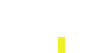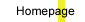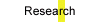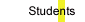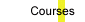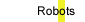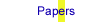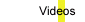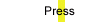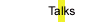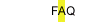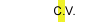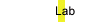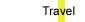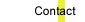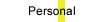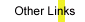## THE CANONICAL DISTORTION MEASURE FOR VECTOR QUANTIZATION AND FUNCTION APPROXIMATION

by Jonathan Baxter

To measure the quality of a set of vector quantization points a means of measuring the distance between a random point and its quantization is required. Common metrics such as the Hamming and Euclidean metrics, while mathematically simple, are inappropriate for comparing natural signals such as speech or images. In this paper it is shown how an environment of functions on an input space X induces a canonical distortion measure (CDM) on X. The depiction "canonical" is justified because it is shown that optimizing the reconstruction error of X with respect to the CDM gives rise to optimal piecewise constant approximations of the functions in the environment. The CDM is calculated in closed form for several different function classes. An algorithm for training neural networks to implement the CDM is presented along with some encouraging experimental results.